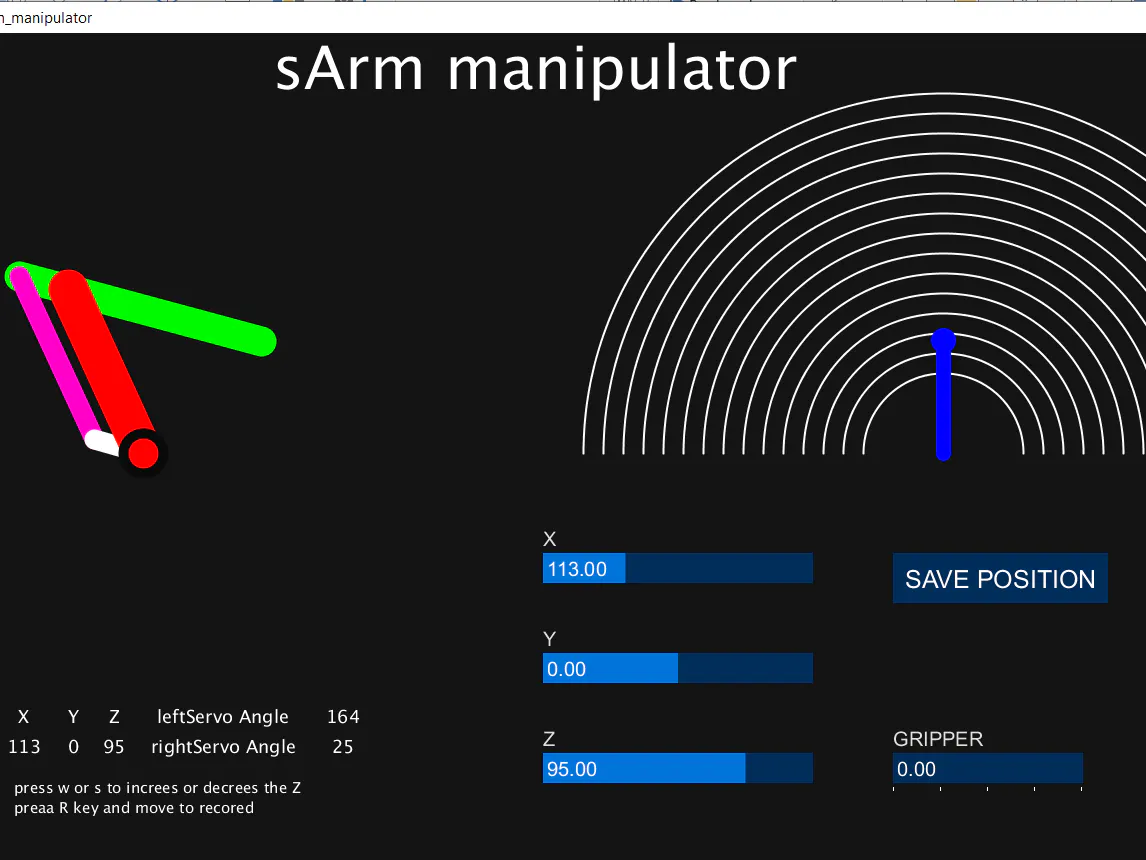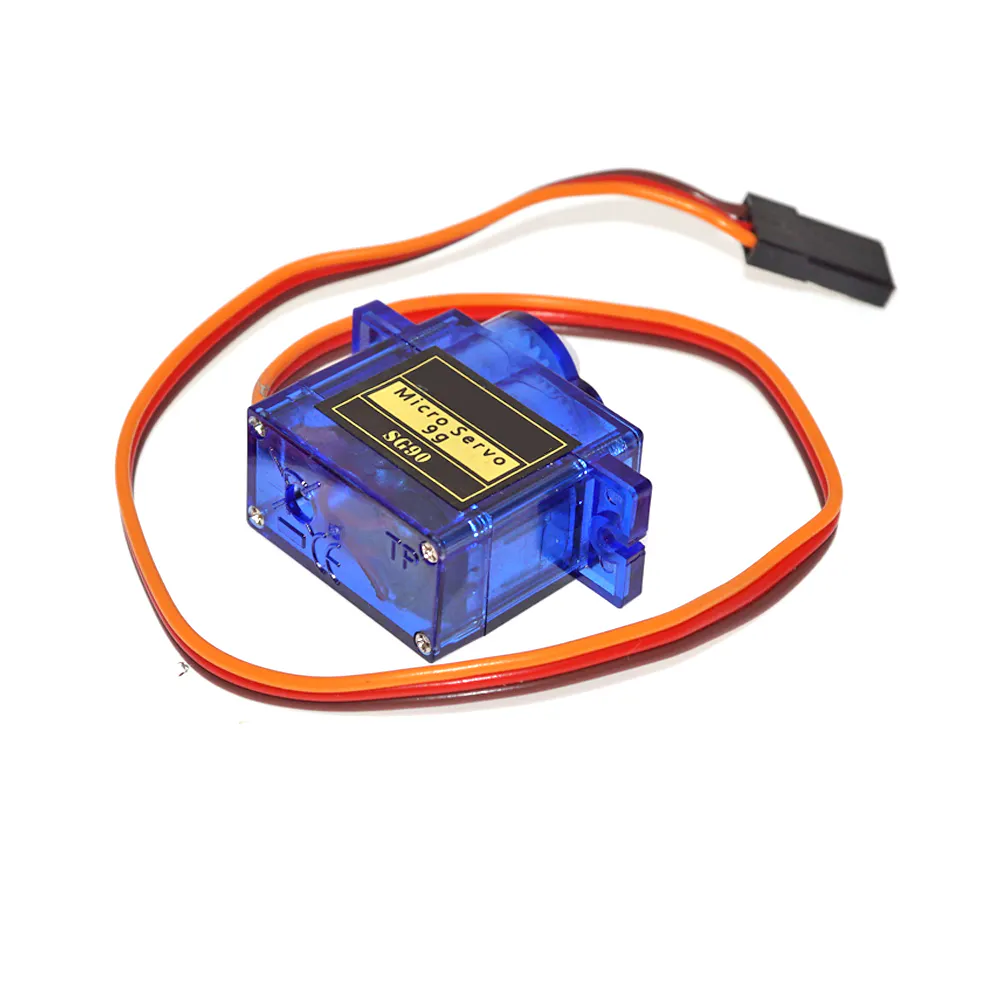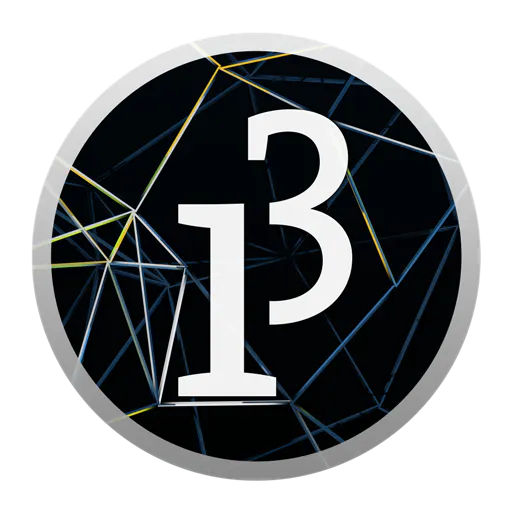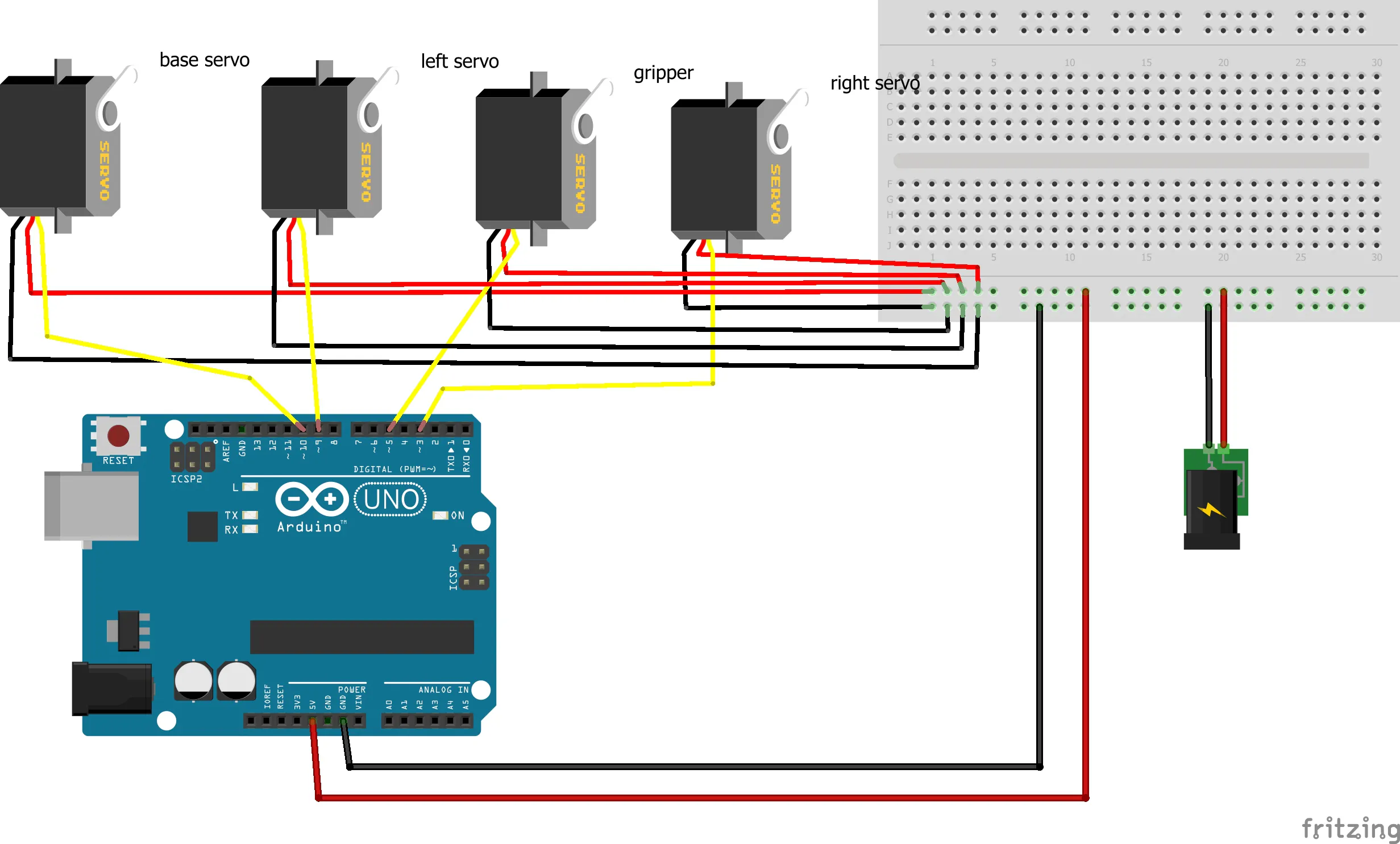Published

# sArm

Robotic Arm Smart Controller System with IoT

IntermediateFull instructions provided252## Things used in this project

### Hardware componentsArduino UNO
×1SG90 Micro-servo motor
×1Jumper wires (generic)
×1

### Software apps and online servicesThe Processing Foundation ProcessingArduino IDEBlynk

## Schematics

### Circuit## Code

### Arduino code

Arduino
```#include <Servo.h>
#include <math.h>

Servo sl, sr, base, gr; // sl - left Servo, sr - right Servo, base - base Servo gr - gripper servo
double l1 = 8, l2 = 8; // measurements of sArm

/////////////////////////////////////////////////////////////////////////////////////////////
void setup() {
//attach the servos
sl.attach(9);
sr.attach(3);
base.attach(10);
gr.attach(5 , 544, 853);

homepos();
}

////////////////////////////////////////////////////////////////////////////////////////////////Initial sArm position.
void homepos() {
mvsl(180, 20);
delay(500);
mvsr(30, 20);
delay(500);
mvb(90, 20);
delay(500);
gr.write(30);
delay(1000);
}

//////////////////////////////////////////////////////////////////////////////////////////////// We use for loops so we can control the speed of the servo
void mvsl( int p, int d) { // to move the left servo
//  p - end position d - delay
// If previous position is bigger then current position
int pp = sl.read(); // set previous position
if (pp > p) {
for ( int j = pp; j >= p; j--) {   // Run servo down
sl.write(j);
delay(d);    // defines the speed at which the servo rotates
}
}
// If previous position is smaller then current position
if (pp < p) {
for ( int j = pp; j <= p; j++) {   // Run servo up
sl.write(j);
delay(d);
}
}
if (pp == p) {
delay(2);
}
}
void mvsr( int p, int d) {// to move the right servo
//  p - end possion d - delay
// If previous position is bigger then current position
int pp = sr.read(); // set previous position
if (pp > p) {
for ( int j = pp; j >= p; j--) {   // Run servo down
sr.write(j);
delay(d);    // defines the speed at which the servo rotates
}
}
// If previous position is smaller then current position
if (pp < p) {
for ( int j = pp; j <= p; j++) {   // Run servo up
sr.write(j);
delay(d);
}
}
if (pp == p) {
delay(2);
}
}
void mvb( int p, int d) {// to move the base servo
//  p - end possion d - delay
// If previous position is bigger then current position
int pp = base.read(); // set previous position
if (pp > p) {
for ( int j = pp; j >= p; j--) {   // Run servo down
base.write(j);
delay(d);    // defines the speed at which the servo rotates
}
}
// If previous position is smaller then current position
if (pp < p) {
for ( int j = pp; j <= p; j++) {   // Run servo up
base.write(j);
delay(d);
}
}
if (pp == p) {
delay(2);
}
}

//open or closs the gripper
//  1 - open 0 - close
void grf( int p) {
int pp = gr.read(); // set previous position
if (p == 1) {
for ( int j = pp; j >= 5; j--) {
gr.write(j);
delay(20);    // defines the speed at which the servo rotates
}
}
if (p == 0) {
for ( int j = pp; j <= 30; j++) {
gr.write(j);
delay(20);
}
}
}

//////////////////////////////////////////////////////////////////////////////////////////////
//Function for inverse kinematics for sArm
// Move end efactor to corsponting (X,Y,Z)
void ikMoveTo(double x, double y, double z) {
double t3 = degrees(atan2(y , x));
double t2 = degrees(acos((pow(x, 2) + pow(y, 2) + pow(z, 2) - pow(l1, 2) - pow(l2, 2)) / (2 * l1 * l2)));
double t1 = degrees(atan(z / (sqrt(pow(x, 2) + pow(y, 2)))) + atan(l2 * sin(radians(t2)) / (l1 + l2 * cos(radians(t2)))));

mvsl((int(180 - (t2 - (t1 - 30)))), 5);
mvsr((int(130 - t1)), 5);
mvb((int(90 + t3)), 5);
}

//////////////////////////////////////////////////////////////////////////////////////////////// Draw a stright line that connect given two points.
void drawLine(int a, int b, int c, int p, int q, int r) {
if (a < p) {
for (int t = a; t <= p; t++) {
double x = t;
double y = (((x - a) * (q - b)) / (p - a)) + b;
double z = (((x - a) * (r - c)) / (p - a)) + c;
ikMoveTo(x, y, z);
delay(10);
}
}
if (a > p) {
for (int t = a; t >= p; t--) {
double x = t;
double y = (((x - a) * (q - b)) / (p - a)) + b;
double z = (((x - a) * (r - c)) / (p - a)) + c;
ikMoveTo(x, y, z);
delay(10);
}
}
if (a == p) {
if (b < q) {
for (int t = b; t <= q; t++) {
double y = t;
double x = (((y - b) * (p - a)) / (q - b)) + a;
double z = (((x - a) * (r - c)) / (p - a)) + c;
ikMoveTo(x, y, z);
delay(10);
}
}
if (b > q) {
for (int t = b; t >= q; t--) {
double y = t;
double x = (((y - b) * (p - a)) / (q - b)) + a;
double z = (((x - a) * (r - c)) / (p - a)) + c;
ikMoveTo(x, y, z);
delay(10);
}
}
if (b == q) {
if (c < r) {
for (int t = c; t <= r; t++) {
double z = t;
double y = (((z - c) * (q - b)) / (r - c)) + b;
double x = (((z - c) * (p - a)) / (r - c)) + a;

ikMoveTo(x, y, z);
delay(10);
}
}
if (c > r) {
for (int t = c; t >= r; t--) {
double z = t;
double y = (((z - c) * (q - b)) / (r - c)) + b;
double x = (((z - c) * (p - a)) / (r - c)) + a;

ikMoveTo(x, y, z);
delay(10);
}
}
if (c == r) {
ikMoveTo(a, b, c);
}
}
}
}
//////////////////////////////////////////////////////////////////////////////////////////////
// Draw a triangle that connect given three points in XYZ space.
void drawTri(int a, int b, int c, int p, int q, int r, int x, int y, int z) {
drawLine(a, b, c, p, q, r);
delay(500);
drawLine(p, q, r, x, y, z);
delay(500);
drawLine(x, y, z, a, b, c);
delay(500);
}
//////////////////////////////////////////////////////////////////////////////////////////////
void loop() {
// program for a sample task, sArm do this again and again
// you can program the sArm with these functions easily

drawTri(10, 0, 5, 17, 0, 13, 17, 0, 0);
delay(1000);
ikMoveTo(10, 1, 10);
delay(1000);
drawLine(10, 0, 0, 10, 0, 9);
delay(10);
grf(1);
delay(1000);
drawLine(10, 0, 9, 10, 0, 0);
delay(10);
grf(0);
delay(1000);
}
```

### Processing Code

Java
```import processing.serial.*;
import controlP5.*;
import cc.arduino.*;

Arduino arduino;
ControlP5 cp5;

float x=113, y = 0, z=95, x1=113, y1, t1, t2, t3;
float  l1=180, l2=200, l3=50; // sArm mesurements
int k=0, k1=0; // index for seaved poses
float sliderX=x, sliderY=y, sliderZ=z, sliderG;
// arraies for save pos
float[] posX = new float;
float[] posY = new float;
float[] posZ = new float;
int rs=0; // run status

void setup() {
size(1440, 840);

cp5 = new ControlP5(this);

println(Arduino.list());
//Select port from the listed array.
//replace  to ,...for selecting a usable open port.
arduino = new Arduino(this, Arduino.list(), 57600);

arduino.pinMode(9, Arduino.SERVO); // left servo
arduino.pinMode(3, Arduino.SERVO); // right servo
arduino.pinMode(10, Arduino.SERVO); // base servo
arduino.pinMode(5, Arduino.SERVO); // gripper

PFont pfont = createFont("Arial", 225, true); // use true/false for smooth/no-smooth
ControlFont font = new ControlFont(pfont, 20);
ControlFont font2 = new ControlFont(pfont, 25);

//X controls
.setPosition(600, height/2+100)
.setSize(270, 30)
.setRange(0, l1+l2-10) // Slider range, corresponds to Joint 1 or theta1 angle that the robot can move to
.setColorLabel(225)
.setCaptionLabel("X")
.setFont(font)
;

//Y controls
.setPosition(600, height/2+200)
.setSize(270, 30)
.setRange(-(l1+l2-50), l1+l2-50) // Slider range, corresponds to Joint 1 or theta1 angle that the robot can move to
.setColorLabel(225)
.setCaptionLabel("Y")
.setFont(font)
;

//Z controls
.setPosition(600, height/2+300)
.setSize(270, 30)
.setRange(-160, 180) // Slider range, corresponds to Joint 1 or theta1 angle that the robot can move to
.setColorLabel(225)
.setCaptionLabel("Z")
.setFont(font)
;

.setPosition(950, height/2+300)
.setSize(190, 30)
.setRange(0, 100)
.setColorLabel(225)
.setFont(font)
.setCaptionLabel("Gripper")
.setNumberOfTickMarks(5)
;

.setPosition(950, 520)
.setSize(215, 50)
.setFont(font2)
.setCaptionLabel("SAVE POSITION")
;

.setPosition(1205, 520)
.setSize(215, 50)
.setFont(font2)
.setCaptionLabel("RUN PROGRAM")
;

.setPosition(1270, 700)
.setSize(135, 40)
.setFont(font)
.setCaptionLabel("(CLEAR)")
;
}

void draw() {
background(20);

PVector m = new PVector(mouseX, mouseY); // position vector of mouse
PVector c = new PVector(200, height/2);  //position vector of new center

pushMatrix();
translate(200, height/2);
PVector c1 = new PVector(800, 0);
m.sub(c);

if (k1>=k) {
k1=0;
}

if (rs==1) { // if run butten is pressed run the seaved possions
x=posX[k1];
y=posY[k1];
z=posZ[k1];
sliderX=x;
sliderZ=z;
sliderY=y;
}
k1++;

// to contrall the side view simulation
if (mousePressed == true && ((sq(200-mouseX) + sq(height/2-mouseY))<=sq(l1+l2)) && mouseX>200) {
x1 = m.x;
z = -m.y;
x = sqrt(sq(x1)-sq(y)) ;
sliderX=x;
sliderZ=z;
}

// to contrall the top view simulation
if (mousePressed == true && ((sq(1000-mouseX) + sq(height/2 - mouseY))<=sq(l1+l2 -5)) && mouseY<height/2) {
y= m.x - 800;
x = -m.y;
x1 = sqrt(sq(y)+sq(m.y)) ;
sliderX=x;
sliderY=y;
}

x=sliderX;
y=sliderY;
z=sliderZ;

//press w or s to increes or decrees the Z
if (keyPressed) {
if (key == 'w' || key == 'W') {
z++;
}
if (key == 's' || key == 'S') {
z--;
}
}

cp5.getController("sliderX").setValue(x);
cp5.getController("sliderY").setValue(y);
cp5.getController("sliderZ").setValue(z);

//Inverse kinematics based on vectos.
t3= atan2(y, x);
t2= acos((sq(x) +sq(y)+sq(z)-sq(l1)-sq(l2))/(2*l1*l2));
t1= (atan2(z, ( sqrt(sq(x)+sq(y)))) + atan2(l2*sin(t2), (l1+l2*cos(t2))));

PVector a = new PVector(l1*cos(-t1), l1*sin(-t1));
PVector b = new PVector();
PVector p = new PVector(y, x);
p.sub(c1);
m.set(x1, -z);
b=PVector.sub(a, m);

stroke(0, 250, 0);
strokeWeight(30);
line(a.x, a.y, a.x+l3*cos(t4), a.y+l3*sin(t4));
b.mult(-1);
line(a.x, a.y, a.x+l2*cos(t5), a.y+l2*sin(t5));
stroke(255, 0, 200);
strokeWeight(20);
line(l3*cos(t4), l3*sin(t4), a.x+l3*cos(t4), a.y+l3*sin(t4));
stroke(255);
line(0, 0, l3*cos(t4), l3*sin(t4));
stroke(255, 0, 0);
strokeWeight(40);
line(0, 0, a.x, a.y);
stroke(10);
ellipse(0, 0, 10, 10);

for (int i=160; i<=2*(l1+l2 - 10); i=i+40) {
stroke(255);
strokeWeight(2);
noFill();
arc(800, 0, i, i, PI, 2*PI);
}

if  (((sq(p.x+800) + sq(p.y))<=sq(l1+l2-5)) && -p.y<0) {
stroke(0, 0, 255);
strokeWeight(15);
line(800, 0, p.x + 1600, -p.y);
ellipse(p.x +1600, -p.y, 10, 10);
}

PVector l = new PVector(1, 0);
l.mult(-1);
float al = PVector.angleBetween(l, b);
//l.mult(-1);
float ar = PVector.angleBetween(l, a);

// move the servos to the positions.
arduino.servoWrite(9, int(degrees(al)) );
arduino.servoWrite(3, int(degrees(ar)) );
arduino.servoWrite(10, int(90 +degrees(t3)) );

//print the texts on the GUI
textSize(18);
textAlign(CENTER);
text("X", -120, 270);
text("Y", -70, 270);
text("Z", -30, 270);
text(int(x), -120, 300);
text(int(y), -70, 300);
text(int(z), -30, 300);
text("leftServo Angle", 80, 270);
text(int(degrees(al)), 200, 270);
text("rightServo Angle", 80, 300);
text(int(degrees(ar)), 200, 300);
textSize(15);
textAlign(LEFT);
text("press w or s to increes or decrees the Z", -130, 340);
text("preaa R key and move to recored", -130, 360);
textSize(60);
text("sArm manipulator", 130, -365);
popMatrix();
//preaa R key and move to recored
if (keyPressed) {
if (key == 'r' || key == 'R') {
posX[k]= x;
posY[k]=y;
posZ[k]=z;
k++;
cp5.getController("savePosition").setCaptionLabel("RECODING");
cp5.getController("savePosition").setColorLabel(#e74c3c);
}
} else {
cp5.getController("savePosition").setCaptionLabel("SAVE POSITION");
cp5.getController("savePosition").setColorLabel(255);
}
}
// seave position with butten
public void savePosition() {
posX[k]= x;
posY[k]=y;
posZ[k]=z;
k++;
}
// Play the seaved positions
public void run() {
if (rs == 0) {
cp5.getController("run").setCaptionLabel("STOP");
cp5.getController("run").setColorLabel(#e74c3c);

rs = 1;
} else if (rs == 1) {
rs = 0;
cp5.getController("run").setCaptionLabel("RUN PROGRAM");
cp5.getController("run").setColorLabel(255);
}
}
// clear the seaved poses
public void clearSteps() {
for (int i =0; i<k; i++) {
posX[i]=posX[k];
posY[i]=posY[k];
posZ[i]=posZ[k];
}
k=0;
posX[k]= 113;
posY[k]=0;
posZ[k]=95;
}
```

## Credits

### sathursan_s

0 projects • 1 follower